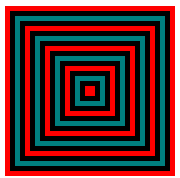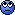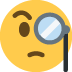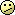# SVG concentric border widths

It’s larger than all the others, how would I make it the same size? While keeping everything even.

The middle is 10px while the outer border lines are all 6px.``````<svg width="170" height="170" viewBox="0 0 170 170">
<rect x="0" y="0" width="170" height="170" fill="red" />
<rect x="5" y="5" width="160" height="160" fill="black" />
<rect x="10" y="10" width="150" height="150" fill="teal" />
<rect x="15" y="15" width="140" height="140" fill="black" />
<rect x="20" y="20" width="130" height="130" fill="red" />
<rect x="25" y="25" width="120" height="120" fill="black" />
<rect x="30" y="30" width="110" height="110" fill="teal" />
<rect x="35" y="35" width="100" height="100" fill="black" />
<rect x="40" y="40" width="90" height="90" fill="red" />
<rect x="45" y="45" width="80" height="80" fill="black" />
<rect x="50" y="50" width="70" height="70" fill="teal" />
<rect x="55" y="55" width="60" height="60" fill="black" />
<rect x="60" y="60" width="50" height="50" fill="red" />
<rect x="65" y="65" width="40" height="40" fill="black" />
<rect x="70" y="70" width="30" height="30" fill="teal" />
<rect x="75" y="75" width="20" height="20" fill="black" />
<rect x="80" y="80" width="10" height="10" fill="red" />
</svg>
``````

That it not what I am seeing.Try removing this…

``width="170" height="170"``

of that pokey jsfidle and you will see that your
assertions are incorrect.

Perhaps it’s just an optical illusion.@coothead yeah I didn’t see anything larger or smaller than the rest eitherYou are young and probably blessed with 20/20 visual acuity.Whereas I am very old and can barely…yes, true lol. But even I… my eyes sometimes wanders off deeply…very, very deeply…“With her one eye in the pot and the other up the chimney.”The Drummer and the Cook

1 Like

I shall read that soon, thank youHi there asasass,

Full Page View:-

Editor View:-

2 Likes

That’s mesmerising, I just wish I could find an app where it would be usefulHi there asasass,

Full Page View:-

Editor View:-

eg. at `40,40` (40 px from top, 40 px from left) there is a `90x90` square. On top of that there is a `80x80` square positioned at `45,45`
The last square is `10x10` hence it appears larger than the thickness of the illusory borders.